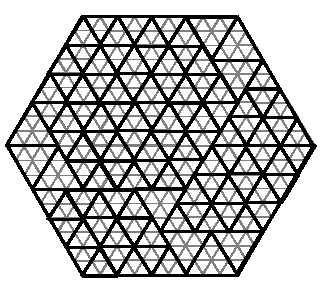Online JudgeProblem SetAuthorsOnline ContestsUser
Web Board
F.A.Qs
Statistical Charts
Problems
Submit Problem
Online Status
Prob.ID:
Register
Authors ranklist
Current Contest
Past Contests
Scheduled Contests
Award Contest
Register
Language:
The Bermuda Triangle
 Time Limit: 2000MS Memory Limit: 32768K Total Submissions: 1760 Accepted: 809

Description

People in the hidden region of the Bermuda Triangle make everything they need in triangular shapes. One day, someone decided to break the rule and bake a hexagonally shaped cake. But as usual, he has to serve the cake in triangular pieces. The pieces are equilateral triangles but in different sizes for different people. He can use as many triangles as needed to cut the cake into pieces, such that nothing remains from the cake. For example, the following figure shows one way that a hexagon with side 9 can be cut into triangles with side 2 and 3. (The cake is cut along the thick lines, thin lines are drawn to show the sizes).Input is a hexagon and triangle types (specified by the length of their sides) and the goal is to decide if the hexagon can be completely divided by the given triangle types.

Input

The first line of the input file contains a single integer t (1 <= t <= 10), the number of test cases, followed by the input data for each test case. Each test case consists of a single line, containing s (1 <= s <= 25), the length of the hexagon's side, followed by n, the number of triangle types (1 <= n <= 10), followed by n integers representing the length of each triangle type's side (between 1 and 25, inclusive).

Output

There should be one output line per test case containing either YES or NO depending on whether the hexagon can be completely divided by the given triangle types.

Sample Input

```3
5 2 2 3
7 2 3 2
13 2 2 3
```

Sample Output

```NO
NO
YES
```

Source

[Submit]   [Go Back]   [Status]   [Discuss]Home PageGo BackTo top